# AP Physics 2 Practice Test 7

### Test Information10 questions18 minutes

1. Which of the following is an isotope of Carbon with 7 neutrons in it?

2.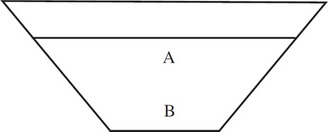A cup of water is shaped as shown. You can say

3.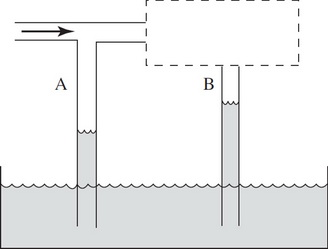A tube with two T branches that have an open end is inserted in a liquid. However, the section of the tube above section B is hidden from view. The hidden section may be wider or narrower. Air is blown through the tube and the water levels rise as shown. You can say

4.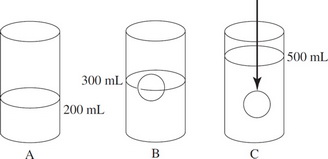In the figure above, you have a cylinder with water in it as shown above in picture A. In picture B you place a ball in that floats. In picture C you physically push the ball under the water to just below the surface. What is the density of the ball?

5. Question below refers to the following diagram: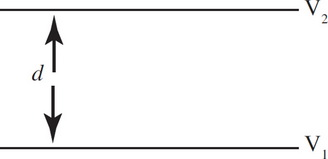Two large flat parallel plates are separated by a distance of d. The lower plate is at a potential of V1 with respect to the ground. The upper plate is at a potential of V2 with respect to the ground.

How much work is done moving a charge Q and mass m from the lower plate to the upper plates?

6. Question below refers to the following diagram:Two large flat parallel plates are separated by a distance of d. The lower plate is at a potential of V1 with respect to the ground. The upper plate is at a potential of V2 with respect to the ground.

The particle on the top plate is now released from rest. The velocity it has as it strikes the bottom plate is given by

7.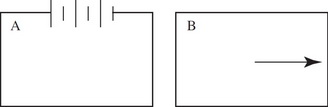Two loops of conducting wire are near each other as shown. Loop A has a battery attached as indicated. Loop B is now pulled away from loop A. What can we say about the direction of the current in each loop as loop B is pulled away as shown?

Loop A     Loop B

8. Question below refers to the following circuit diagram.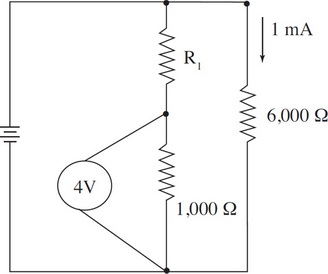What is the voltage across R1 ?

9. Question below refers to the following circuit diagram.What is the current coming out of the battery?

10.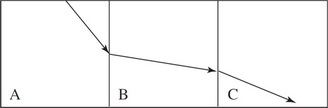In the figure above, a ray of light travels through three different regions (mediums) as shown. List the three regions in order of increasing index of refraction.